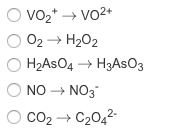# Problem: Which transformation could take place at the anode of an electrochemical cell?

###### FREE Expert Solution

To determine the reaction that can take place in the anode we have to follow the relationship below.

anode → oxidation → oxidation number increases

The rules for oxidation states are as follows:

A. General Rules:

1. For an atom in its elemental form (Zn, Cl2, C(graphite), etc.)    O.S. = 0

2. For an ion (Li+, Al3+, etc.)                                                       O.S. = charge

B. Specific Rules:

1. Group 1A              O.S. = +1

2. Group 2A              O.S. = +2

3. Hydrogen              O.S. = +1, with nonmetals

O.S. = –1 with metals and boron

4. Fluorine                 O.S. = –1

5. Oxygen                  O.S. = –1 in peroxides (X2O2, X = Group 1A)

O.S. = –1/2 in superoxides (XO2, X = Group 1A)

O.S. = –2 in other compounds

6. Group 7A               O.S. = –1 (except when bonded to O)

96% (82 ratings)###### Problem Details

Which transformation could take place at the anode of an electrochemical cell?